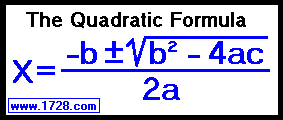Cubic Equation Calculator Quartic Equation CalculatorTo see how the Quadraric Formula is derived, click here Quadratic Equation Calculator
 Input MUST have the format: AX2 + BX + C = 0 EXAMPLE: input the equation    2X2 + 4X -30 = 0 as: A= 2  B= 4   C= -30 click E N T E R and your answers should be 3 and -5.
Note: Do not enter commas.
A= B= C=

X1=

X2=

Here's the answer in fractional form:

X=

Other Information